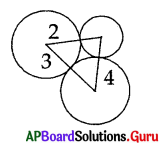Practice the AP 10th Class Maths Bits with Answers Chapter 9 Tangents and Secants to a Circle on a regular basis so that you can attempt exams with utmost confidence.

## AP State Syllabus 10th Class Maths Bits 9th Lesson Tangents and Secants to a Circle with Answers

Question 1.
The number of parallel tangents of a circle with a given tangent is
A) 1
B) 2
C) 0
D) infinite
A) 1

Question 2.
PA and PB are two tangents drawn to a circle with centre O from an external point P. If ∠APB = 30°, then ∠AOB =
A) 60°
B) 90°
C) 70°
D) 150°
D) 150°Question 3.
Radius of a circle with centre ‘O’ is 5 cm. P is a point at a distance of 3 cm from ‘O’. Then the number of tangents that can be drawn to the circle is ………………
A) 1
B) 2
C) 0
D) 3
C) 0

Question 4.
Angle made by the minutes – hand in a clock during a period of 20 minutes is ……………….
A) 120°
B) 20°
C) 360°
D) 90°
A) 120°

Question 5.
From the adjacent figure, ∠APB = 40°, then ∠AOB = ……………….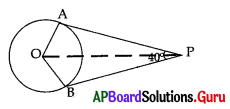A) 110°
B) 140°
C) 80°
D) 160°
B) 140°

Question 6.
The length of a tangent to a circle from a point P is 12 cm and the radius of the circle is 5 cm, then the distance from point P to the centre of the circle is ……………..
A) 11 cm
B) 10 cm
C) 13 cm
D) 14 cm
C) 13 cm

Question 7.
Perimeter of a sector is ………………….. units.
A) $$\frac{l \mathrm{r}}{2}$$
B) $$\frac{\mathbf{x}^{0}}{360^{0}}$$ × πr2
C) 2 (l + r)
D) l + 2r
D) l + 2r

Question 8.
Angle of a sector is 60° and radius is 14 cm, then its area is ……………….. cm2.
A) 100.6
B) 102.67
C) 111.6
D) 98.66
B) 102.67

Question 9.
The longest chord in a circle is …………………
A) diameter
C) arc
D) perimeter
A) diameterQuestion 10.
A circle can have …………….. parallel tangents.
A) 1
B) 3
C) 2
D) 4
C) 2

Question 11.
How many tangent lines can be drawn to a circle from a point outside the circle?
A) 1
B) 2
C) 3
D) 4
B) 2

Question 12.
Two circles with centres A and B touches each other at the point C. If AC = 8 cm and AB = 3 cm, then area of shaded region is…………………..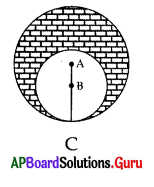A) 13π
B) 5π
C) 39π
D) 8π
C) 39π

Question 13.
The C.S.A. and T.S.A. of a cylinder are in the ratio 2 : 3. Its T.S.A. is 924 cm2 then
its volume is ……………….. cm3.
A) 2156
B) 1256
C) 1265
D) 2516
A) 2156

Question 14.
The minutes hand of a clock length is 10.5 cm. Then area swept by it in 10 minutes is ……………….. cm2.
A) 115.5
B) 57.75
C) 11.55
D) 575.5
B) 57.75

Question 15.
Two circles of radius 15 cm and 13 cm intersect at A and B, such that $$\overline{\mathbf{A B}}$$ = 24 cm. The distance between their centres is …………… cm
A) 28
B) 14
C) 18
D) 16
B) 14

Question 16.
The distance between the two parallel tangents of a circle whose radius is 12 cm is ………………. cm.
A) 14
B) 24
C) 28
D) 36
B) 24Question 17.
The end points of diameter of a circle are (2, 4) and (-3, -1). The radius of the circle is ……………..
A) $$\frac{5 \sqrt{2}}{2}$$
B) 5√2
C) 3√2
D) $$\frac{5 \sqrt{2}}{\pm 2}$$
A) $$\frac{5 \sqrt{2}}{2}$$

Question 18.
The area of a circle is 220 cm2. Then the area of square inscribed in it is……………..
A) 49 cm2
B) 70 cm2
C) 140 cm2
D) 150 cm2
C) 140 cm2

Question 19.
Area of segment of a circle …………………….
A) area of sector
B) area of corresponding sector / area of corresponding triangle
C) 2(l + r)
D) area of segment sector + πr2
B) area of corresponding sector / area of corresponding triangle

Question 20.
If TP and TQ are two tangents to a circle with centre O so that ∠POQ = 110°, then ∠PTQ is equal to
A) 60°
B) 70°
C) 80°
D) 90°
B) 70°

Question 21.
What is the area of the shaded region in the figure ? In which two circles with centres A and B touch each other at the point C, if AC = 8 cm and AB = 3 cm, is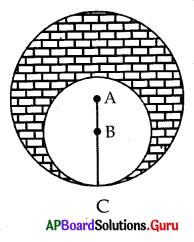A) 24π cm2
B) 39π cm2
C) 11π cm2
D) 57π cm2
A) 24π cm2

Question 22.
If all the sides of a parallelogram touch a circle, then the parallelogram is
A) a square
B) a rhombus
C) a rectangle
D) None
C) a rectangle

Question 23.
PQ is chord of length 8 cm of a circle of radius 5 cm. The tangents at P and Q intersect at a point T. Then the length of TP is
A) $$\frac{10}{3}$$cm
B) $$\frac{25}{3}$$cm
C) $$\frac{20}{3}$$cm
D) $$\frac{16}{3}$$cm
D) $$\frac{16}{3}$$cmQuestion 24.
The number of circles passing through three collinear points in a plane is
A) 1
B) 0
C) 9
D) 12
B) 0

Question 25.
The number of pairs of parallel tangents to a circle is
A) 2
B) 4
C) 1
D) infinitely many
D) infinitely many

Question 26.
The length of the tangent to a circle with centre O and radius = 6 cm from a point P outside tlie circle such that OP = 10 cm is
A) 6 cm
B) 8 cm
G) 4 cm
D) 5 cm
B) 8 cm

Question 27.
If PA and PB are the lengths of tangents drawn from an external point P to a circle, then
A) PA ≠ PB
B) PA > PB
C) PA < PB
D) PA = PB
D) PA = PB

Question 28.
The area of the sector, whose radius is 7 cm with angle 60°, is
A) 52.66 cm2
B) 25.66 cm2
C) 62.56 cm2
D) 65.62 cm2
B) 25.66 cm2

Question 29.
In the adjacent figure, x =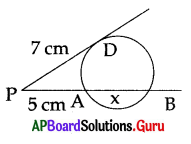A) 5.8 cm
B) 6.8 cm
C) 4.8 cm
D) 3.8 cm
C) 4.8 cm

Question 30.
In the figure, x =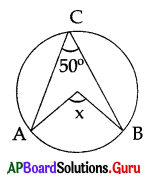A) 100°
B) 90°
C) 110°
D) 80°
A) 100°Question 31.
How many tangents can be drawn to a circle from a point outside the circle ?
A) 1
B) 2
C) 3
D) None
B) 2

Question 32.
A secant intersects the circle at ……………… point (s).
A) single
B) two
C) three
D) none
B) two

Question 33.
The tangent PQ at a point P of a circle of radius 5 cm meets a line through the centre O at a point Q so that OQ = 12 cm. Then the length of the tangent is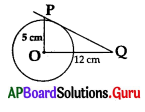A) 13 cm
B) $$\sqrt{119}$$ cm
C) 14 cm
D) 11 cm
B) $$\sqrt{119}$$ cm

Question 34.
A parallelogram circumscribing a circle is a
A) trapezium
B) rhombus
C) square
D) rectangle
B) rhombus

Question 35.
If the radius of a circle is 4 cm and the sector angle is 30°, then the area of the sector is
A) $$\frac{3 \pi}{4}$$sq.cm
B) 3π
C) 4π sq.cm
D) $$\frac{4 \pi}{3}$$sq.cm
D) $$\frac{4 \pi}{3}$$sq.cm

Question 36.
In the figure below, which of the following are tangents to the circle?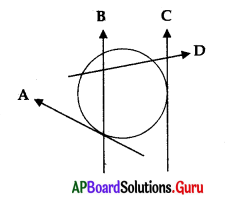A) A, B, C
B) A, D
C) A, C
D) A, B, D
C) A, C

Question 37.
In the figure below, OP = 3 cm and OB = 5 cm. Then AB =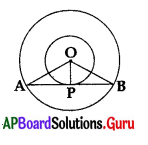A) 8 cm
B) 9 cm
C) 4 cm
D) 10 cm
A) 8 cmQuestion 38.
In the figure if ∠APB = 60° and OP = 10 cm, then PA = …………….. cm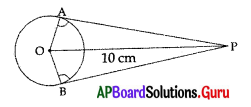A) 5
B) 5√2
C) 5√3
D) 20
C) 5√3

Question 39.
The angle between a tangent to a circle and the radius drawn at the point of contact is ………………
A) 60°
B) 30°
C) 45°
D) 90°
D) 90°

Question 40.
A line which intersects the given circle at two distinct points is called a
A) tangent
B) transversal
C) direct line
D) secant
D) secant

Question 41.
The number of pair of tangents can be drawn to a circle, which are parallel to each other, are ……………….
A) 0
B) 2
C) 4
D) infinite
B) 2

Question 42.
The number of tangents that can be drawn to a circle from an external point is ……………….
A) 0
B) 3
C) 2
D) 1
C) 2

Question 43.
If PA and PB are tangents to a circle of radius 5 cm, then their lengths are ……………. cm.
A) 5, 13
B) 13, 13
C) 12, 12
D) 13, 12
C) 12, 12

Question 44.
The angle between a tangent and the radius drawn at the point of contact is
A) 60°
B) 30°
C) 90°
D) 45°
C) 90°

Question 45.
From a point Q, the length of the tangent to a circle is 24 cm, and the distance of Q from the centre is 25 cm, then the radius of the circle is
A) 7 cm
B) 12 cm
C) 15 cm
D) 24 .5 cm
A) 7 cmQuestion 46.
The length of the tangent drawn from a point 17 cm away from the centre of a circle of radius 8 cm is
A) 25 cm
B) 9 cm
C) 15 cm
D) 8.5 cm
C) 15 cm

Question 47.
If the length of a tangent to a circle of radius 6 cm is 8 cm, then the distance from which the tangent is drawn to the centre of the circle is
A) 7 cm
B) 8 cm
C) 9 cm
D) 10 cm
D) 10 cm

Question 48.
Two concentric circles are of radii 5 cm and 3 cm. Length of the chord of the larger circle which touches the smaller circle is
A) 8 cm
B) 5 cm
C) 7. 5 cm
D) 9 cm
A) 8 cm

Question 49.
If tangents PA and PB from a point P to a circle with centre ‘O’ are inclined to each other at angle of 80°, then ∠POA =
A) 50°
B) 60°
C) 70°
D) 80°
A) 50°

Question 50.
AP and AQ are two tangents of a circle with centre ‘O’ and ∠POQ = 60°, then ∠OPQ =
A) 30°
B) 45°
C) 60°
D) 90°
C) 60°

Question 51.
If two tangents to a circle of radius 3 cm are inclined to each other at angle of 60°, then length of each tangent =
A) 6 cm
B) 3√3 cm
C) 3 cm
D) $$\frac{3 \sqrt{3}}{4}$$cm
B) 3√3 cm

Question 52.
The area of a sector of a circle of radius 7 cm and central angle 60° is …………………….
A) $$\frac{77}{3}$$
B) $$\frac{77}{6}$$
C) $$\frac{77}{9}$$
D) $$\frac{154}{5}$$
A) $$\frac{77}{3}$$Question 53.
In the figure if BP = 5cm,QC = 7cm and AR = 6 cm, then AB + BC + CA =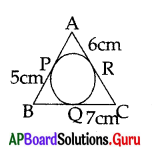A) 18 cm
B) 36 cm
C) 25 cm
D) 30 cm
B) 36 cm

Question 54.
The parallelogram circumscribing a circle is a ……………………
A) Trapezium
B) Triangle
C) Rectangle
D) Square
C) Rectangle

Question 55.
Cyclic rhombus is a …………………
A) Parallelogram
B) Square
C) Trapezium
D) Rectangle
B) Square

Question 56.
In the adjacent figure if quadrilateral PQRS circumscribes a circle, then PB + SD =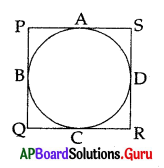A) SR
B) PR
C) QS
D) PS
D) PS

Question 57.
In the figure ∠AOB =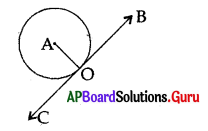A) 80°
B) 90°
C) 100°
D) 20°
B) 90°

Question 58.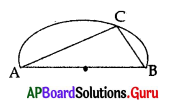In the figure ∠ACB =
A) 60°
B) 80°
C) 90°
D) 45°
C) 90°

Question 59.
The set of all points which are equidistant from a fixed point is called a
A) line
B) ray
C) circle
D) triangle
C) circleQuestion 60.
The line segment joining any two points oq a circle is called
B) diameter
C) chord
D) tangent
C) chord

Question 61.
The set of all points which are equidistant from a fixed point is a circle, then the fixed distance is called
A) diameter
Cj centre
D) chord

Question 62.
A line meeting a circle only in one point is called a
A) secant
B) tangent
C) chord
D) arc
B) tangent

Question 63.
A line, which intersects a circle in two distinct points, is called
A) secant
B) tangent
C) chord
D) arc
A) secant

Question 64.
The point at which the tangent touches the circle is called
A) point of intersection
B) centre of the circle
C) point of contact
D) external point of the circle
C) point of contact

Question 65.
Number of tangents that can be drawn at point on a circle is
A) 1
B) 2
C) 3
D) infinite
A) 1

Question 66.
Number of tangents that can be drawn through a point inside a circle is
A) 0
B) 1
C) 2
D) infinite
A) 0Question 67.
How many tangents a circle can have?
A) 1
B) 2
C) 0
D) infinite
D) infinite

Question 68.
Number of parallel tangents that a circle can have at the most is
A) 1
B) 2
C) 3
D) infinite
B) 2

Question 69.
A line drawn through the end of a radius and perpendicular to it, is a …………….. to the circle.
A) secant
B) tangent
C) chord
D) arc
D) arc

Question 70.
The tangent at any point of a circle is to the …………………. radius through the point of contact.
A) equal
B) similar
C) parallel
D) perpendicular
D) perpendicular

Question 71.
The length of a tangent from a point d cm away from the centre of a circle of radius ‘r’ is
A) $$\sqrt{d^{2}-r^{2}}$$
B) $$\sqrt{\mathrm{d}^{2}+\mathrm{r}^{2}}$$
C) $$\sqrt{\mathrm{dr}}$$
D) $$\sqrt{\mathrm{d}+\mathrm{r}}$$
A) $$\sqrt{d^{2}-r^{2}}$$

Question 72.
Two concentric circles are of radii a and b (a>b). Length of the chord of the larger circle which touches the small circle is
A) 2$$\sqrt{a^{2}-b^{2}}$$
B) $$\sqrt{a^{2}-b^{2}}$$
C) 2$$\sqrt{a^{2}+b^{2}}$$
D) $$\sqrt{a^{2}+b^{2}}$$
A) 2$$\sqrt{a^{2}-b^{2}}$$

Question 73.
If AP and AQ are two tangents to a circle with centre O so that ∠POQ = 110°, then ∠PAQ = ………………..
A) 60°
B) 70°
C) 80°
D) 90°
B) 70°Question 74.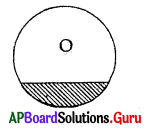A) Minor segment
B) Major segment
C) Centre
D) Sector
A) Minor segment

Question 75.
Number of chords of a circle is
A) 1
B) 2
C) 3
D) infinite
D) infinite

Question 76.
Number of tangents that can be drawn from outside the circle is
A) 0
B) 1
C) 2
D) infinite
C) 2

Question 77.
The distance between two parallel tangents to a circle of diameter 8 cm is
A) 8
B) 6
C) 4
D) 2
A) 8

Question 78.
The limit of a secant line of a circle is
A) chord
B) diameter
C) tangent
D) secant
A) chord

Question 79.
A tangent PA touches a circle with centre ‘O’ at A, then
A) ∠OPA = 90°
B) ∠POA = 90°
C) ∠OAP = 45°
D) ∠OAP = 90°
D) ∠OAP = 90°

Question 80.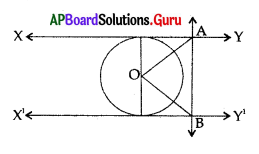In the figure XY and XY are two parallel tangents to a circle with centre ‘O’ and other tangent AB with point of contact ‘C’ intersecting XY at A and X’Y’ at B, then ∠AOB =
A) 80°
B) 100°
C) 90°
D) 60°
C) 90°

Question 81.
Two tangents TP and TQ are drawn to a circle with centre ‘O’ from an external point T. The ∠PTQ =
A) ∠OPQ
B) 2∠OPQ
C) ∠POQ
D) 2∠POQ
B) 2∠OPQ

Question 82.
In the adjacent figure PA and PB are tangents to a circle with centre ‘O’ then ∠OPA =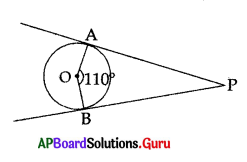A) 45°
B) 50°
C) 70°
D) 35°
A) 45°Question 83.
In the adjacent figure if PA = 4 cm, BP = 3 cm and AC = 11 cm then BC =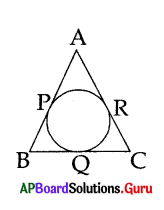A) 15 cm
B) 14 cm
C) 7 cm
D) 10 cm
D) 10 cm

Question 84.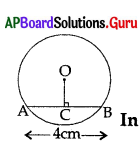In the figure AB = 4cm, then BC = ……………….. cm
A) 6
B) 4
C) 2
D) 8